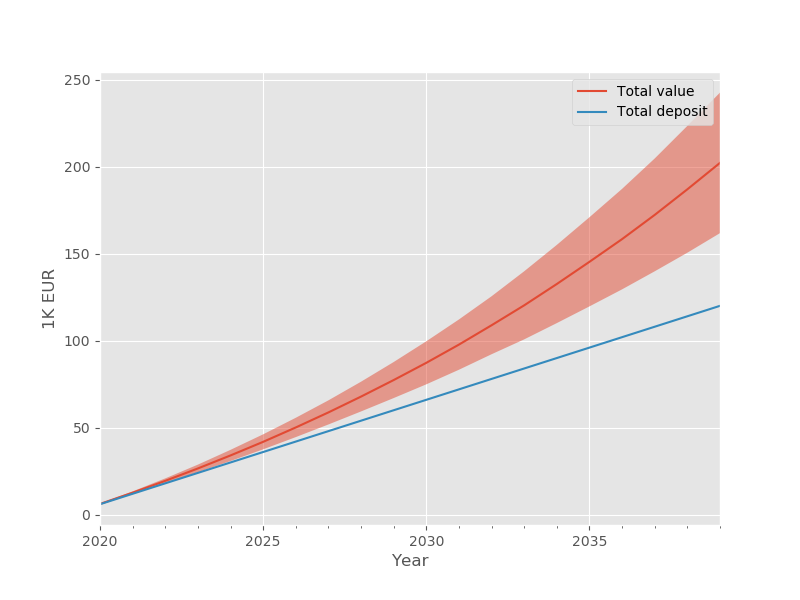# Compound Interest: what is it?

###### PUBLISHED ON NOV 16, 2019 — FINANCE

On the path of educating myself on personal finance, I encountered the term compound interest or compounding effect.

## A well-known reality, for some

Compounding is a term that describes how consistently investing a relatively small portion of money in market shares and/or bonds will, on the long term (typically 10-20 years), provide massively greater value than the bare amount one has invested.

This is a very well known concept to people familiar with Economics and Finance but relatively unknown to the laymen in the street, who may be struggling to get to the end of the month (“Investing? What?”).

## A practical example

Here is an example of compounding at work. If every month I put EUR 500 into market shares (let’s say in a portfolio loosely representing the US market), in 20 years I will have accumulated about EUR 183000, out of EUR 120000 that I have deposited, which totals a 68% return on my overall investment (assuming that the market will have on average a 5% annual return — a historically fair assumption).
This holds under the following conditions:

• I keep depositing the given amount every month;
• all the accumulated interest is reinvested;
• I don’t sell, also known as a hold position.

## Understanding the magic formula with Python

The first time I read about it I was a bit skeptical. How am I creating so much value, seemingly out of nothing?

In an effort to understand how compounding works, I wrote a small python function to simulate a plausible scenario and see for myself how this effect works.

The question is: in twenty years and with average market conditions, how much will be the return on investment (ROI) from putting 500 Euros in my portfolio every month?

I put this logic in a Python function, adding normal random noise to simulate the fluctuation of markets around the 5% average. Here’s what it does:

1. Add 500 to the pot;
2. Generate the interest rate;
3. Calculate interest;
4. Add interest to current amount;
5. Calculate total current amount;
6. Repeat for every month until reaching 20 years.
``````import numpy as np
import pandas as pd

def calc_comp_interest_mini(
initial_sum=0,
deposit=500,
yearly_comp_frequency=12,
n_years=20,
yearly_interest=.05,
starting_date = "2020-01-01",
random_seed=42
):
np.random.seed(random_seed)
date = pd.to_datetime(starting_date) + pd.tseries.offsets.MonthEnd(1)
data = []
final_sum = initial_sum

for i in range(yearly_comp_frequency * n_years):
current_total = final_sum + deposit
simulated_rate = np.random.normal(
loc=yearly_interest,
scale=.12,
) / yearly_comp_frequency
current_total_interest = simulated_rate * current_total
final_sum = (
current_total
+ current_total_interest
)

data.append([
date,
current_total,
current_total_interest,
simulated_rate,
final_sum,
])
date = (
date
+ pd.to_timedelta(1, unit='days')
+ pd.tseries.offsets.MonthEnd(1)
)
data = pd.DataFrame(
data,
columns=[
'month',
'principal',
'interest',
'rate',
'total',
]
).set_index('month')
return data
``````

I have run the code about 4000 times in order to have a reliable average.

At year 20, the numbers are the following:

• The value of the portfolio is just shy of 202000 Euros;
• The total return on the 120000 investment is therefore almost 82000, i.e. a 68+% increase in value.

Here’s what it looks like.The graph also shows the 95% confidence interval, based on a 12% standard deviation. However, random gaussian fluctuations are probably not a good model for financial markets. I would consider this just as a simplified demonstration of the compounding effect.

Investing is a very complex topic, but this little experiment made me reflect on the magnitude of the compound effect on long-term investments and on the importance of investing early.